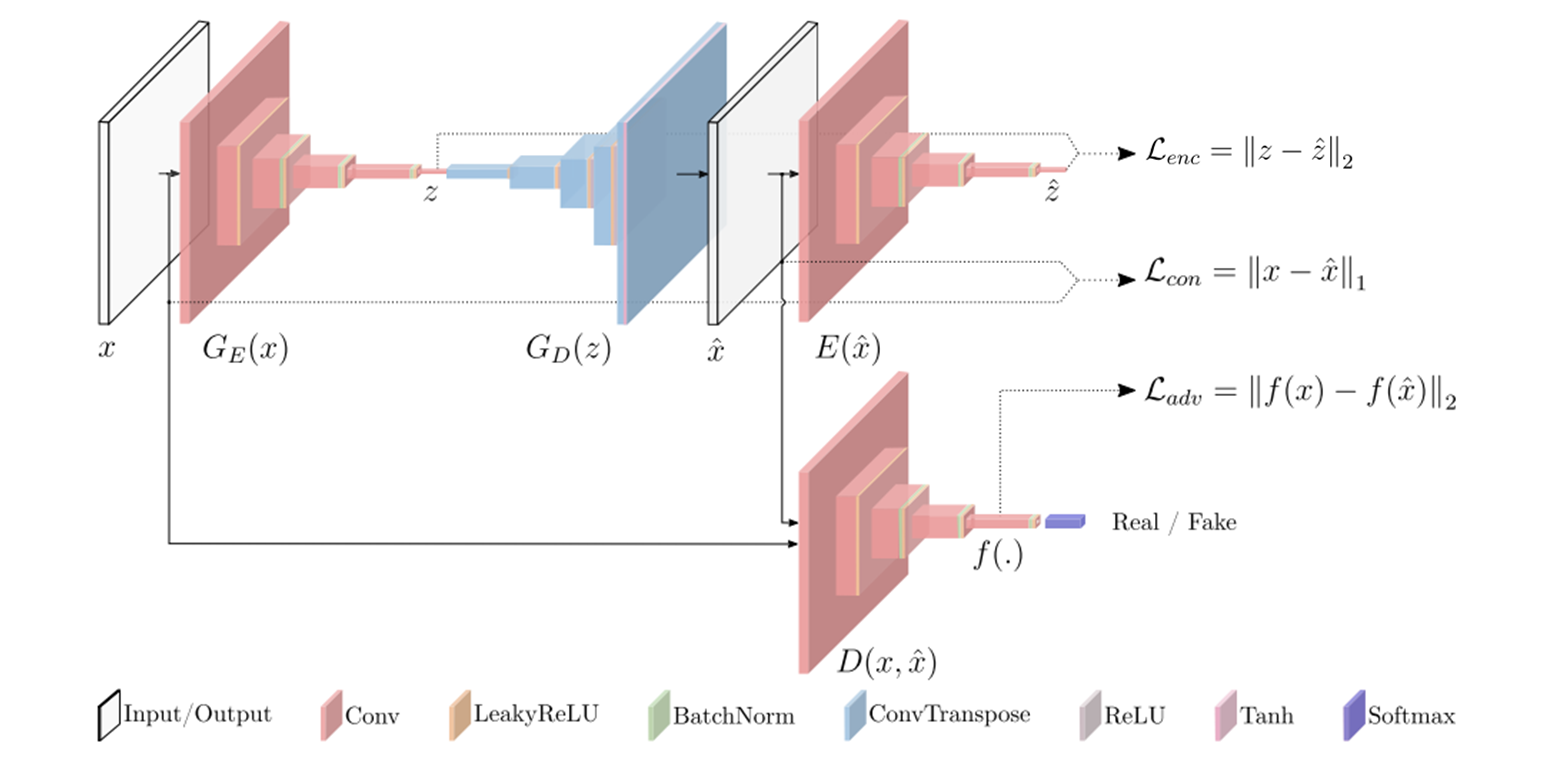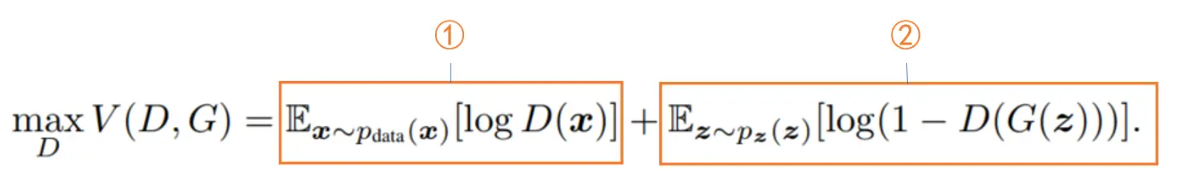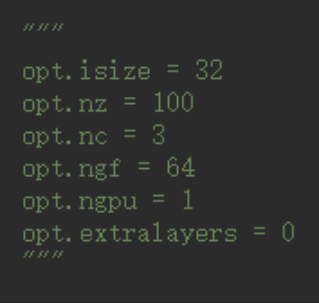# 對抗生成網路GAN系列——GANomaly原理及原始碼解析

theme: fancy highlight: atelier-dune-dark

🍊作者簡介：禿頭小蘇，致力於用最通俗的語言描述問題

🍊近期目標：寫好專欄的每一篇文章

🍊支援小蘇：點贊👍🏼、收藏⭐、留言📩

# 對抗生成網路GAN系列——GANomaly原理及原始碼解析

## 寫在前面

​  在前面，我已經介紹過好幾篇有關GAN的文章，連結如下：

​  這篇文章我將來為大家介紹GANomaly，論文名為：Semi-Supervised Anomaly Detection via Adversarial Training。這篇文章同樣是實現缺陷檢測的，因此在閱讀本文之前建議你對使用GAN網路實現缺陷檢測有一定的瞭解，可以參考上文連結中的和。

​  準備好了嗎，嘟嘟嘟，開始發車。🚖🚖🚖

## GANomaly原理解析

【閱讀此部分前建議對GAN的原理及GAN在缺陷檢測上的應用有所瞭解，詳情點選寫在前面中的連結檢視，本篇文章我不會再介紹GAN的一些先驗知識。】

### GANomaly結構

​  這部分為大家介紹GANomaly的原理，其實我們一起來看下圖就足夠了：圖1 GANomaly結構圖

​  我們還是先來對上圖中的結構做一些解釋。從直觀的顏色上來看，我們可以分成兩類，一類是紅色的Encoder結構，一類是藍色的Decoder結構。Encoder主要就是降維的作用啦，如將一張張圖片資料壓縮成一個個潛在向量；相反，Decoder就是升維的作用，如將一個個潛在向量重建成一張張圖片。按照論文描述的結構來分，可以分成三個子結構，分別為生成器網路G，編碼器網路E和判別器網路D。下面分別來介紹介紹這三個子結構：

• 生成器網路G

​  生成器網路G由兩個部分組成，分別為編碼器$G_E(x))$和解碼器$G_D(z)$，其實這就是一個自動編碼器結構，主要用來學習輸入x的資料分佈並重建影象${\hat x}$。我們一個個來看，先看$G_E(x)結構$，假設我們的輸入x維度為$\mathbb{R}^{C×H×W}$，經過$G_E(x)結構$後，變成一個向量$z$，其維度為$\mathbb{R}^d$。【$G_E(x)$具體結構很簡單啦，這裡就不詳細介紹了。我會在原始碼解析部分給出，大家肯定一看就會。】接著我們來看$G_D(z)$結構，它會將剛剛得到的向量z上取樣成$\hat x$，$\hat x$的維度和$x$一致，都為$\mathbb{R}^{C×H×W}$。關於$G_D(Z)$結構也很簡單，其主要用到了轉置卷積，對於轉置卷積不瞭解的可以看部落格瞭解詳情。生成器網路G就為大家介紹完了，是不是發現很簡單呢。總結下來就兩步，第一步讓輸入x通過$G_E(x)$得到z，第二步讓z通過$G_D(Z)$變成$\hat x$。這兩步也可以用一步表示，即$\hat x=G(x)$。

​  思來想去我還是想在這裡給大家丟擲一個問題，我們傳統的GAN是怎麼通過生成器來構建假影象的呢？和GANomaly有區別嗎？其實這個問題的答案很簡單，大家都稍稍思考一下，我就不給答案了，不明白的評論區見吧！！！🥂🥂🥂

• 編碼器網路E

​  編碼器網路E的作用是將生成器得到的$\hat x$壓縮成一個向量$\hat z$，是不是發現和生成器網路中的$G_E(x)$很像呢，其實呀，它倆的結構就是完全一樣的，生成的$\hat z$ 和$\hat x$ 的維度一致，這是方便後面的損失比較。

• 判別器網路D

​  判別器網路D和我們之前介紹DCGAN時的結構是一樣的，都是將真實資料$x$和生成資料$\hat x$輸入網路，然後得出一個分數。

### GANomaly損失函式

​  GANomaly的損失函式分為兩部分，第一部分是生成器損失，第二部分為判別器損失，下面我們分別來進行介紹：

• 生成器損失函式

​  生成器損失函式又由三個部分組成，分別如下：

我還是直接上公式吧，如下：

​        $$L_{adv}=E_{x \sim px}||f(x)-E_{x \sim px}f(G(x))||_2$$

這個公式對應圖一中的$L_{adv}=||f(x)-f(\hat x)||_2$🍵🍵🍵這個損失函式應該很好理解，在前面介紹的GAN網路都有提及，$f(*)$表示判別器網路某個中間層的輸出。這個損失函式的作用就是讓兩張影象$x和\hat x$儘可能接近，也就是讓生成器生成的圖片更加逼真。

• Contextual Loss

同樣的，直接來上公式，如下：

​        $$L_{con}=E_{x \sim px}||x-G(x)||_1$$

這個公式對應圖一中的$L_{con}=||x-\hat x||_1$🍵🍵🍵這個函式其實也是要讓兩張影象$x和\hat x$儘可能接近。至於這裡為什麼用的是L1範數而不是L2範數，作者在論文中說這裡使用L1範數的效果要比使用L2範數的效果好，這屬於實驗得到的結論，大家也不用過於糾結。

• Encoder Loss

話不多說，上公式，如下：

​        $$L_{enc}=E_{x \sim px}||G_E(x)-E(G(x))||_2$$

這個公式對應圖一中的$L_{enc}=||z-\hat z||_2$🍵🍵🍵這裡的損失函式在我看來主要作用就是讓我們在推理過程中的效果更好，這裡就像AnoGAN中不斷搜尋最優的那個z的作用。

如果大家這裡讀過cycleGAN的論文的話，可能會覺得這個損失函式有點類似cycleGAN中的迴圈一致性損失。我覺得這篇文章的思想可能借鑑了cycleGAN中的思想，感興趣的可以去閱讀一下，非常有意思的一篇文章！！！🥃🥃🥃

$$L=w_{adv}L_{adv}+w_{con}L_{con}+w_{enc}L_{enc}$$

在論文提供的原始碼中，預設$w_{con}=50,w_{adv}=w_{enc}=1$。

• 判別器損失函式

判別器的損失函式就和原始GAN一樣，如下：【不清楚的點選☞☞☞瞭解詳情】python self.l_bce = nn.BCELoss() # Real - Fake Loss self.err_d_real = self.l_bce(self.pred_real, self.real_label) self.err_d_fake = self.l_bce(self.pred_fake, self.fake_label)

# NetD Loss & Backward-Pass self.err_d = (self.err_d_real + self.err_d_fake) * 0.5 

### GANomaly測試階段

​  在上一小節，為大家介紹了GANomaly的損失函式，這是在測試階段使用的。GANomaly針對的是異常檢測任務，在測試階段我們會對輸入的資料進行評分，根據評分的結果來判定輸入是否異常。在GANomaly中使用的評分函式就是我們上一小節介紹的Encoder Loss，對於一個測試資料x，用$A(x)$表示其異常得分，則：

​          $$A(x)=||G_E(x)-E(G(x))||_2$$

​  這裡大家需要注意以下，論文中$A(x)$的表示式使用的是L1範數，但是從我閱讀論文提供的原始碼來看，程式碼中使用的是L2範數。這裡保持和原始碼一致，使用L2範數。程式碼中關於此部分的描述如下：

python

# latent_i表示G_E(x)，latent_o表示E(G(x))。torch.pow(m,2)=m^2

error = torch.mean(torch.pow((latent_i-latent_o), 2), dim=1) 

## GANomaly原始碼解析

​  這裡直接使用論文中提供的原始碼地址：GANomaly原始碼🌱🌱🌱

### GANomaly模型搭建

​  其實通過我前文的講解，不知道大家能否感受到GANomaly模型其實是不復雜的。需要注意的是在介紹GANomaly結構時我們將模型分為了三個子結構，分別為生成器網路G、編碼器網路E、判別器網路D。但是在程式碼中我們將生成器網路G和編碼器網路E合併在一塊兒了，也稱為生成器網路G。

​  下面我給出這部分的程式碼，大家注意一下這裡面的超引數比較多，為了方便大家閱讀，我把這裡用到超引數的整理出來，如下圖所示：python """ Network architectures. """

# pylint: disable=W0221,W0622,C0103,R0913

import torch import torch.nn as nn import torch.nn.parallel from options import Options

def weights_init(mod): """ Custom weights initialization called on netG, netD and netE :param m: :return: """ classname = mod.class.name if classname.find('Conv') != -1: mod.weight.data.normal_(0.0, 0.02) elif classname.find('BatchNorm') != -1: mod.weight.data.normal_(1.0, 0.02) mod.bias.data.fill_(0)

class Encoder(nn.Module): """ DCGAN ENCODER NETWORK """

def __init__(self, isize, nz, nc, ndf, ngpu, n_extra_layers=0, add_final_conv=True):
super(Encoder, self).__init__()
self.ngpu = ngpu
assert isize % 16 == 0, "isize has to be a multiple of 16"

main = nn.Sequential()
# input is nc x isize x isize
nn.Conv2d(nc, ndf, 4, 2, 1, bias=False))
nn.LeakyReLU(0.2, inplace=True))
csize, cndf = isize / 2, ndf     # csize=16,cndf=64

# Extra layers
for t in range(n_extra_layers):
nn.Conv2d(cndf, cndf, 3, 1, 1, bias=False))
nn.BatchNorm2d(cndf))
nn.LeakyReLU(0.2, inplace=True))

while csize > 4:
in_feat = cndf
out_feat = cndf * 2
nn.Conv2d(in_feat, out_feat, 4, 2, 1, bias=False))
nn.BatchNorm2d(out_feat))
nn.LeakyReLU(0.2, inplace=True))
cndf = cndf * 2
csize = csize / 2

# state size. K x 4 x 4
nn.Conv2d(cndf, nz, 4, 1, 0, bias=False))

self.main = main

def forward(self, input):
if self.ngpu > 1:
output = nn.parallel.data_parallel(self.main, input, range(self.ngpu))
else:
output = self.main(input)

return output


class Decoder(nn.Module): """ DCGAN DECODER NETWORK """ def init(self, isize, nz, nc, ngf, ngpu, n_extra_layers=0): super(Decoder, self).init() self.ngpu = ngpu assert isize % 16 == 0, "isize has to be a multiple of 16"

    cngf, tisize = ngf // 2, 4    #cngf=32 ,tisize=4
while tisize != isize:
cngf = cngf * 2
tisize = tisize * 2

main = nn.Sequential()
# input is Z, going into a convolution
nn.ConvTranspose2d(nz, cngf, 4, 1, 0, bias=False))
nn.BatchNorm2d(cngf))
nn.ReLU(True))

csize, _ = 4, cngf
while csize < isize // 2:
nn.ConvTranspose2d(cngf, cngf // 2, 4, 2, 1, bias=False))
nn.BatchNorm2d(cngf // 2))
nn.ReLU(True))
cngf = cngf // 2
csize = csize * 2

# Extra layers
for t in range(n_extra_layers):
nn.Conv2d(cngf, cngf, 3, 1, 1, bias=False))
nn.BatchNorm2d(cngf))
nn.ReLU(True))

nn.ConvTranspose2d(cngf, nc, 4, 2, 1, bias=False))
nn.Tanh())
self.main = main

def forward(self, input):
if self.ngpu > 1:
output = nn.parallel.data_parallel(self.main, input, range(self.ngpu))
else:
output = self.main(input)
return output


## 判別器網路結構

class NetD(nn.Module): """ DISCRIMINATOR NETWORK """

def __init__(self, opt):
super(NetD, self).__init__()
model = Encoder(opt.isize, 1, opt.nc, opt.ngf, opt.ngpu, opt.extralayers)
layers = list(model.main.children())

self.features = nn.Sequential(*layers[:-1])
self.classifier = nn.Sequential(layers[-1])

def forward(self, x):
features = self.features(x)
features = features
classifier = self.classifier(features)
classifier = classifier.view(-1, 1).squeeze(1)

return classifier, features


## 生成器網路結構

class NetG(nn.Module): """ GENERATOR NETWORK """

def __init__(self, opt):
super(NetG, self).__init__()

self.encoder1 = Encoder(opt.isize, opt.nz, opt.nc, opt.ngf, opt.ngpu, opt.extralayers)
self.decoder = Decoder(opt.isize, opt.nz, opt.nc, opt.ngf, opt.ngpu, opt.extralayers)
self.encoder2 = Encoder(opt.isize, opt.nz, opt.nc, opt.ngf, opt.ngpu, opt.extralayers)

def forward(self, x):
latent_i = self.encoder1(x)
gen_imag = self.decoder(latent_i)
latent_o = self.encoder2(gen_imag)
return gen_imag, latent_i, latent_o




### GANomaly損失函式

​  我們在理論部分已經介紹了GANomaly的損失函式，那麼在程式碼上它們都是一一對應的，實現起來也很簡單，如下：

python

## 定義L2 Loss

self.l_adv = l2_loss self.l_con = nn.L1Loss() self.l_enc = l2_loss

self.err_g_adv = self.l_adv(self.netd(self.input), self.netd(self.fake)) self.err_g_con = self.l_con(self.fake, self.input) self.err_g_enc = self.l_enc(self.latent_o, self.latent_i) self.err_g = self.err_g_adv * self.opt.w_adv + \ self.err_g_con * self.opt.w_con + \ self.err_g_enc * self.opt.w_enc 

​  上述程式碼為GANomaly生成器損失函式程式碼，判別器的損失函式程式碼已經在理論部分為大家介紹了，這裡就不在贅述了。🍄🍄🍄

### 小結

​  這裡我並沒有很詳細的為大家解讀程式碼，但是把一些關鍵的部分都給大家介紹了。會了這些其實你完全可以自己實現一個GANomaly網路，或者對我之前在Anogan中的程式碼稍加改造也可以達到一樣的效果。論文中提供的原始碼感興趣的大家可以自己去除錯一下，程式碼量也不算多，但有的地方理解起來也有一定的困難，總之大家加油吧！！！🌼🌼🌼

## 參考連結